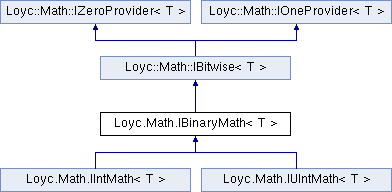Enhanced C# Language of your choice: library documentation
Loyc.Math.IBinaryMath< T > Interface Template Reference

Provides additional bit-oriented integer operations. More...

Source file:
Inheritance diagram for Loyc.Math.IBinaryMath< T >:## Remarks

Template Parameters
 T An integer or integer-based types.

## Public Member Functions

Shl (T a, int amount)
Shifts 'a' left by the specified number of bits. More...

Shr (T a, int amount)
Shifts 'a' right by the specified number of bits. More...

int CountOnes (T a)
Returns the number of '1' bits in 'a'. More...

int Log2Floor (T a)
Returns the floor of the base-2 logarithm of x. e.g. 1024 -> 10, 1000 -> 9 More...Public Member Functions inherited from Loyc.Math.IBitwise< T >
And (T a, T b)

Or (T a, T b)

Xor (T a, T b)

Not (T a)Properties inherited from Loyc.Math.IZeroProvider< T >
Zero` [get]`
Returns the "zero" or additive identity of this type. More...Properties inherited from Loyc.Math.IOneProvider< T >
One` [get]`
Returns the "one" or identity value of this type. More...

## ◆ CountOnes()

 int Loyc.Math.IBinaryMath< T >.CountOnes ( T a )

Returns the number of '1' bits in 'a'.

## ◆ Log2Floor()

 int Loyc.Math.IBinaryMath< T >.Log2Floor ( T a )

Returns the floor of the base-2 logarithm of x. e.g. 1024 -> 10, 1000 -> 9

The return value is int.MinValue for an input of zero (for which the logarithm is technically undefined.)

## ◆ Shl()

 T Loyc.Math.IBinaryMath< T >.Shl ( T a, int amount )

Shifts 'a' left by the specified number of bits.

A shift amount A negative shift amount produces undefined results

## ◆ Shr()

 T Loyc.Math.IBinaryMath< T >.Shr ( T a, int amount )

Shifts 'a' right by the specified number of bits.